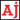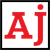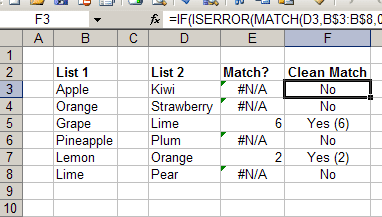# .css-t1tid{-webkit-text-decoration:none;text-decoration:none;background-image:none;text-shadow:none;}Reviews. Info. Tutorials.

# Compare lists in Excel using the MATCH function

Learn how to use the Excel MATCH function to compare lists.
June 30, 2010

Using the `MATCH` function in Excel and you can find out whether a value in one list exists in another.

## MATCH

The `MATCH` function accepts 3 parameters:

1. `lookup_value`

• The value you want to find.
2. `lookup_array`

• The list that you want to search through.
3. `match_type`

• Optional and specifies if you want an exact match or a less/greater than match.

`MATCH` will try to find the `lookup_value` you specify in the list you specify (`lookup_array`) and return the relative position in the list if it finds a match. The relative position means that if your list starts at A5 and the MATCH function finds a match with the first value in your list, it will return a 1 not a 5. So it doesn't return the row it found the match in, just the position within the list.

For `match_type`, unless you're working with numbers then you will usually want to set match_type to 0, which means only find exact matches. If you're trying to match text then this is your best bet. Another thing to note is that `MATCH` will return the relative position of the first match it finds, so if you have duplicate values in the list, `MATCH` will only return the position of the first one.

## ExampleUsing the MATCH function in Excel to compare two lists

The figure above shows a spreadsheet with two lists (List 1 & List 2). The formula for cell E3 in the Match? column is `=MATCH(D3,B\$3:B\$8,0)`. This tells Excel to lookup the value in D3 (Kiwi) and check to see if it is in List 1 which goes from B3 to B8. In this case it doesn't find a match so it returns `#N/A`. However, in this example it does find two matches, Lime and Orange at positions 6 and 2 in the list. The formula in cell E5 is `=MATCH(D5,B\$3:B\$8,0)` which does find a match and returns a 6.

Note: Make sure you include the `\$` when specifying the list to look in, in this case `B\$3:B\$8`. This will keep Excel from automatically changing the list when you copy and paste the formula to find the other values in List 2.

### Clean it up (Optional)

You'll notice in column F under Clean Match it says No if it didn't find a match, and Yes with the position in brackets if it did find a match. To do this you can use the `IF` and `ISERROR` function along with `MATCH` to make it a little cleaner. The formula in cell F3 looks like this: `=IF(ISERROR(MATCH(D3,B\$3:B\$8,0)),"No","Yes (" & MATCH(D3,B\$3:B\$8,0) & ")")`. It's a little longer and more complicated but if we break it down you'll see what is happening. The `IF` function has 3 parts:

1. What you want to check
2. What to do if it's `True`
3. What to do if it's `False`.

In this case we're checking if `ISERROR(MATCH(D3,B\$3:B\$8,0))` is `True` or `False`. We already know what the `MATCH` function does, and the `ISERROR` function just checks to see if the `MATCH` function returns an error (i.e. no match) or a number. If `MATCH` returns `#N/A` then `ISERROR` will be `True`, otherwise it will be `False`.

Now, if `ISERROR` is `True` (i.e. we didn't find a match) then the `IF` function will return the value `"No"`. If `ISERROR` is `False` (i.e. we found a match) then the `IF` function will return the value `"Yes (" & MATCH(D3,B\$3:B\$8,0) & ")"`. This is just the text Yes ( and then we get the position of the lookup value using the MATCH function and close it off with more text, in this case " )", and this is why we got the value Yes(6) when looking for Lime in list 1.

It isn't necessary to do this but sometimes it looks a lot nicer to have a specific result when you find or don't find a match.